Reach Us+44-1522-440391
Money Demand in China: A Banking Approach | OMICS International
International Journal of Economics & Management Sciences
All submissions of the EM system will be redirected to Online Manuscript Submission System. Authors are requested to submit articles directly to Online Manuscript Submission System of respective journal.

# Money Demand in China: A Banking Approach

Peng Zhou*

Lecturer in Economics, Cardiff University, United Kingdom

*Corresponding Author:
Peng Zhou
Lecturer in Economics, D47, Aberconway Building
Colum Drive, Cardiff University, CF10 3EU
United Kingdom
Tel: 0044-7528223250
E-mail: [email protected]

Received Date: November 11, 2016; Accepted Date: November 17, 2016; Published Date: November 21, 2016

Citation: Zhou P (2016) Money Demand in China: A Banking Approach. Int J Econ Manag Sci 5: 381. doi: 10.4172/2162-6359.1000381

Copyright: © 2016 Zhou P. This is an open-access article distributed under the terms of the Creative Commons Attribution License, which permits unrestricted use, distribution, and reproduction in any medium, provided the original author and source are credited.

Visit for more related articles at International Journal of Economics & Management Sciences

#### Abstract

Traditional money demand function is based on partial equilibrium model, which only focuses on the liquidity market. Though working quite well in practice, the empirical results of these models are not robust due to probable misspecifications and omission of important factors. This paper employs the latest development in general equilibrium model, especially banking approach, as the theoretical methodology. This new approach emphasizes the more and more significant role of banking sector in a developing monetary economy like China. It is shown that this model behaves better in theoretical plausibility and empirical robustness. On the other hand, this paper also uses various advanced time series econometrics as the empirical methodology to improve the power of estimations and statistical tests.

#### Keywords

Money demand; General equilibrium; Banking; Time series; China

E41; E44; G21

#### Introduction

China has been enjoyed a fast economic growth in the last three decades. One of the important reasons is its financial reform and development in banking sector. More and more foreign banks and nonstate- owned banks participate into the competition in banking industry. The productivity of banking sector keeps growing dramatically during the golden decade between the 1997 Asian financial crisis and the 2008 financial crisis. Undoubtedly, the development in financial system will have significant effects on the monetary economy, especially on money demand. This paper strives to incorporate this important factor into the study of money demand in China.

The key feature of this paper is to combine the latest development in theoretical literature and empirical literature. In practice, traditional partial equilibrium models do work quite well to match data in earlier studies. However, the empirical evidence in this paper shows that they are not robust to empirical approaches and money aggregates. The estimated elasticities of money demand change dramatically in value and significance. It shows a probable misspecification in the traditional model. It might be because it ignores the role of banking sector. Latest development in theoretical monetary economics brings in the attention to banking sector. This new strand of models is the basis for this study of money demand. On the other hand, in addition to the traditional econometric tools, I also employ some advanced time series techniques to improve the reliability of the empirical results. Different approaches also enable me to conduct robustness check.

To develop a new methodology based on general equilibrium model, a comprehensive theoretical literature review is done in Section 2 in a generic framework and historical link. Some empirical literatures are also reviewed on both partial equilibrium models and general equilibrium models in Section 3. Based on the previous two sections, the theoretical and empirical methodologies are developed in Section 4. Section 5 presents the model developed from banking approach general equilibrium model, resulting in the money demand function. To compare and contrast this resulting money demand function, a traditional money demand function is also used. Section 6 describes the data problems and related solutions, while Section 7 displays the empirical results with implications and explanations. Section 8 summarizes and concludes.

#### Theoretical Literature Review

The monetary economics is mainly developed based on the assumption of developed economy. On the demand side, money demand is the desired holding of money balances in the form of cash or zero interest bank deposits. People demand for money due to several motives, around which the models of money demand are developed. On the supply side, money supply is assumed to be sticking to some rule (e.g. Taylor rule) so that the monetary economy is always in equilibrium, i.e. they do not talk about transition but only focus on steady state or balanced growth path equilibria.

In terms of origin, monetary economics has evolved from two different streams: the quantity theory, which was a part of the Classical theory, and the Keynesian theory. In terms of methodology, monetary economic models can be divided into partial equilibrium models and general equilibrium models. The former focuses on money market treating the other parts of the economy as exogenous, while the later incorporates all the markets with micro foundation. Partial equilibrium models are still influential today because of its power on explaining empirical data.

Partial equilibrium models

It is often assumed that real money balances are positively related to income and inversely related to the opportunity cost of holding money. These influences can be referred to the two most important motives of money demand, i.e. transactions motive and speculative motive. These provide the original ideas for money demand function.

Based on the Transactions Motive, money demand focuses on the liquidity provided by money. A typical partial equilibrium money demand function usually has a form:

M=P.L (R,y)

M is money demand, P is the price level, R is the nominal interest rate, and y is the real output. The function L(R,y) is called liquidity function. These 1 models provide significant empirical insights into money demand.

Based on the Speculative Motive, money demand focuses on the potential return on various assets (including money) as an additional motive. Keynes  stressed that the choice between money and bonds also depends on both the current nominal interest rate and the expected future interest rate. Tobin  argued that money could be regarded as a risk free asset with zero return and money demand can then be determined according to the portfolio management approach.

Origins of monetary economics

Classical money demand theory: The quantity theory is the name given to the ideas on the determination of the price level from the middle of 18th century to the publication of Keynes  “The General Theory”. This was an evolutionary tradition with several distinct approaches to the role of money, e.g. Fisher, Pigou and Wicksell. Despite different representations, they share a common feature, i.e. a change in the money supply (equal to money demand in equilibrium) in the economy causes a proportionate change in the price level. To obtain this proposition, quantity theory is based on the quantity equation: Mtvt=Ptyt. Take logs on both hand sides, and then take derivative with respect to time: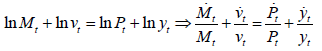Fisher assumed that the velocity vt is constant and output level is in full employment (i.e. steady state), so the growth rate of money supply is equal to the growth rate of price level. Pigou claimed that the velocity vt is a function of interest rate R and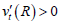. Wicksell, as a Swedish monetary economist within the Classical tradition, has a very distinctive and different treatment of the quantity theory. He focuses on the transmission mechanism relating changes in the money supply to changes in the price level for a pure credit economy in the short run.

Keynesian money demand theory: Classical quantity theory was criticized by Keynes , who asserted the usual absence of fully employment in the economy, and argued that output and velocity depend on the money supply. Keynes summarized three motives of holding money:

Transactions motive

The transactions-motive, i.e. the need of cash for the current transaction of personal and business exchanges .

The transactions motive is further separated into an "income motive" to bridge the interval between the receipt of income and its disbursement by households, and a "business motive" to bridge the interval between payments by firms and their receipts from the sale of their products. Hence, the transactions money demand rises as the nominal income, Ptyt, increases. The transactions motive is essentially consistent with Classical quantity theory.

Precautionary motive

To provide for contingencies requiring sudden expenditure and for unforeseen opportunities of advantageous purchases, and also to hold an asset of which the value is fixed in terms of money .

The individual’s precautionary money demand rises with the uncertainty of future income and consumption needs and purchases.

Speculative motive

The speculative-motive, i.e. the object of securing profit from knowing better than the market what the future will bring forth .

The individual has to make a decision between holding bonds and holding money, with a speculative motive to maximize the maturity value (equal to principal plus interest). Since individuals tend to differ in their views on the future of the interest rate, some would expect an increase in bond prices (“bull”) and choose to increase their bond holdings, while others would expect a decrease in bond prices (“bear”) and reduce their bond holdings. As a result, the speculative money demand increases as the bond price rises, or conversely, as the interest rate falls. Due to the “animal spirit” when people make this choice, money demand would be quite unstable.

Monetarist money demand theory

Friedman  restated the proposition of quantity theory as “money matters” rather than the more specific statement that “changes in money supply will cause proportional changes in the price level”. The main role of quantity theory is limited to a money demand theory. He treated money as a form of asset in consumer’s utility function and as a form of capital in firm’s production function. In this sense, Friedman’s main concern is the speculative motive rather than transactions motive. On the supply side, Friedman asserted that the determinants of the money supply are independent of those of the money demand.

Friedman also argued that money demand and velocity function are highly stable, so monetary policy has a strong and reliable impact on the economy. This is different from the early Keynesian viewpoints that these functions are volatile and that the monetary policy could not be a reliable stabilization policy, so fiscal policy is strongly preferred. The two opinions were merged to the Neoclassical-Keynesian Synthesis in the 1960’s, i.e. the popularity of the IS-LM model for the macroeconomic analysis of the impact of monetary as well as fiscal policy on aggregate demand.

Constant semi-interest elasticity of money demand: In the light of the original ideas developed by Classical, Keynesian and Monetarist, some popular partial equilibrium models are developed. Cagan  explanation of the post-World War I and World War II European hyperinflations was an amazingly successful work. His specification of the money demand function can be rewritten as: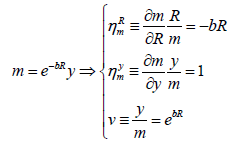Here, b is the "semi-interest elasticity" of money demand, because the magnitude of the interest elasticity rises as the nominal interest rate R goes up. In other words, the consumer’s sensitivity to the inflation tax rises as the inflation tax rate rises, causing the consumer to substitution away from the use of money increasingly more. Rising price elasticity from a rising tax rate is a standard result in public finance literature. Therefore, the success of the Cagan model is that it treats inflation as a tax in a way that is consistent with fiscal tax theory.

From another perspective, if we define the income velocity of money as v≡y/m, then the velocity rises at an increasing rate as the nominal interest rate rises. An example for this money demand function is shown below Graph 1.

Constant interest elasticity of money demand: These models, based on Baumol-Tobin framework, are more suitable for the scenarios when inflation is not significantly high, as opposed to Cagan model.

Baumol  assumes that people have a steady flow of income over the period (partial equilibrium), which can be either deposited in bank to earn nominal interest rate R or held as cash without any interest. However, in order to buy consumption goods, the consumer needs to go to the bank and take out money, which induces a trip cost ‘b’ each time. If M is the amount withdrawn each time, then M/2 is the average holdings of money, i.e. nominal money demand (Graph 2).

Rational consumers choose the optimal money demand by minimizing the total cost, equal to the opportunity cost of interest R (M/2) plus the cost of banking b(y/m):

Min TC=R (M/C)+b (Y/M)

Take derivative of the objective function with respect to the control variable M: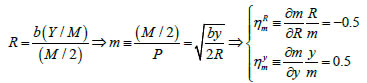The first conclusion is that the marginal cost of money (the foregone interest R) should be equal to the marginal cost banking (the change in the total cost of banking b(Y/M) divided by the change in money holding M/2). The second conclusion is that the real money demand m takes a square root form. It is positively correlated with real income and cost of banking while negatively correlated with the cost of interest. The third conclusion is that this model gives a constant interest elasticity of money demand ηRm=-0.5 and constant income elasticity of money demand ηym=-0.5.

There are mainly two drawbacks on this model. Firstly, this money demand function is not well defined at the Friedman optimum when R=0, where the money demand is infinite. Secondly, the income elasticity is usually considered to be around 1 rather then 0.5, because in any balanced growth equilibrium, money demand needs to stay proportional to consumption and output, i.e. these variables all grow at the same rate. Should that not be the case, the velocity of money would go to zero or infinity. On the other hand, however, some argue that this is exactly how money demand should be analysed because of technological evolution. Woodford argues this as why the economy is moving to a moneyless world and that the velocity of money continues to rise for ever. There is little empirical support for these arguments and most studies still find income elasticity around 1.

Tobin money demand: Money can be treated as a risk free asset when people allocate their wealth. It is a so-called asset approach to money demand. Money is desirable for its zero risk but undesirable for its zero return. Hence, the optimal portfolio between money and bond considers the trade-off between risk and expected return. Tobin  formalized Keynes’ speculative motive of money demand.

General equilibrium models

Money in the utility function (MIUF) model: The central idea is that there is a marginal condition along which the consumer makes the decision of how much money to hold, i.e. the marginal cost equals the marginal benefit of money. The marginal cost of money is the foregone interest from having to carry around money for use in exchange during the period. This opportunity cost is equal to the higher one between the bank savings account interest rate and the government bond interest rate. The marginal benefit of money is the key way in which the monetary economies differ. The benefit could be that money facilitates the exchange or reduces the shopping time. However, abstracting from the precise nature of the benefit, the MIUF simply states that money gives utility.

Static MIUF model: Samuelson assumes that consumer maximizes the utility in a static fashion: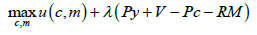The first order conditions give the marginal rate of substitution (MRS) between money and consumption is equal to the nominal interest rate: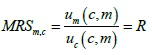Assume that the utility function has a form u(c,m) = ln c + blnm, then the MRS becomes: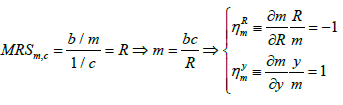The first problem of this model is that the money demand of this form shows a type of “money super neutrality”, i.e. the changes in m have no effect on other variables in the economy. However, this does not hold in general. The second problem is that the interest elasticity of money demand ηRm is too close to −1 while empirical data suggests close to -0.5. A more fundamental problem is that the money demand is not well defined at the Friedman optimum, i.e. R=0.

Dynamic MIUF models: In the dynamic model, the consumer maximizes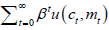, subject to a series of period budget constraints: Ptyt+Vt=Ptct + (Mt+1-Mt). We can equivalently rewrite all the period budget constraints by one "intertemporal budget constraint" using no-Ponzi game condition, i.e. the sum of discounted real value of income should be equal to the sum of discounted real value of expenditure. The consumer’s problem is: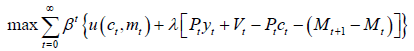The FOCs are combined with government’s budget constraint in steady state equilibrium, i.e. Vt=Mt+1-Mt, to obtain the solution.

On the one hand, we can derive the Intertemporal Condition, which implies that the market discount rate is equal to the subjective discount rate in steady state:

r=ρ

On the other hand, we can also derive the Intratemporal Condition in steady state: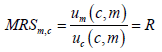This condition is the same as that in Samuelson, i.e. the MRS between money and goods is equal to the price ratio between money (R) and consumption (1). This is because the condensed budget constraint in this dynamic problem is exactly the same as that in the static problem, i.e. yt=ct. The money demand and elasticities can be derived once specific utility function form is given. In steady state, consumption is constant over time and price is growing at a constant inflation rate π.

As a special case, Lucas specifies the utility function as: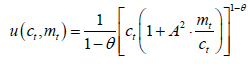Substitute this utility function into the general conclusions above to obtain the money demand and elasticities with a constant interest elasticity feature: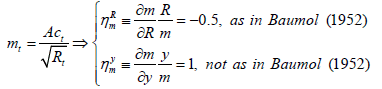Note that the money demand m always enters as part of the ratio m/c because the velocity of money is assumed to be a stable fraction in the equilibrium. Lucas discusses how this specification is not realistic at low nominal interest rates in the sense that Friedman optimum does not hold. To deal with this problem, he considers an example in which government consumes a fraction of resources and the resulting optimal nominal interest rate is above 0.

Another example is Eckstein and Leiderman, where the utility function is specified as:

u (ct,mt)=? (ct)+mt (B-Dlnmt).

Substitute this utility function into the general conclusion to obtain the money demand and elasticities with a constant semi-interest elasticity feature: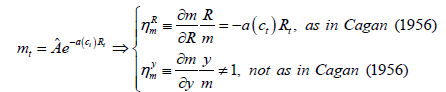Here,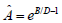is a constant and a (ct)=?′ (ct)/D. This gives an interest elasticity of money demand, which will rise in magnitude with R as in Cagan. However, the income/consumption elasticity of money demand is different from the standard result as in Cagan.

Cash-in-advance model: Transactions motive is the main purpose of holding money when money is the only means of exchange. Cash- In-Advance (CIA) model assumes that good can only be bought by money and the balance of money is determined in previous period. The CIA constraint is also called Clower constraint.

Lucas assumes that there is no capital, and then the consumer’s problem is: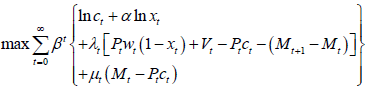Again, the budget constraint can be condensed in steady state by the fact that the government budget constraint holds: Vt=Mt+1-Mt.

On the one hand, the intertemporal condition in steady state, i.e. Euler equation, can be derived from the FOCs, just like in MIUF models. Since all the variables in the condition are constant in steady state, the time subscripts are omitted:

r=ρ

On the other hand, combine the FOCs with respect to ct and Mt+1 to obtain the intratemporal condition in steady state: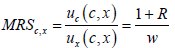This condition says, in steady state, the MRS between consumption and leisure should be equal to the price ratio between goods (1+R) and leisure (W). The price of goods is equal to (1+R) because there is an extra opportunity cost of interest for using money to buy goods. Combine with the budget constraints to obtain the final solution in steady state: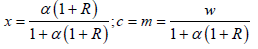Hence, leisure x is positively related with R, while consumption C is negatively related with R, i.e. consumption goes to leisure if the effective goods price rises.

Cash-credit model: In practice, people can purchase goods by cash or by credit rather than by cash only. To model this substitute in transactions, Cash-Credit model is developed.

Lucas and Stokey assume there are two types of goods in terms of the method of purchase: c1t is cash good purchased by cash and c2t is credit good purchased by credit. The price of c1t is 1+Rt, while the price of c2t is 1. To maximize the utility function u(c1t, c2t, xt), the consumer has to trade-off between the extra opportunity cost and the utility loss of cash good. The consumer’s problem now becomes: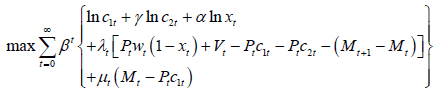Combine the FOCs in st eady state to get the MRS between c1t, c2t and xt: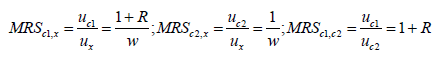The first result is exactly the same as the one in Lucas Cash-in- Advance model. The second result has an intuitive interpretation that the MRS between c2t and x is equal to the price ratio between them. The last result shows that the MRS between c1t and c2t is also equal to the price ratio between them.

Shopping time model: As another way of modelling the money based on transactions motive, shopping time model, is developed by McCallum, Goodfriend and, Gavin and Kydland, Lucas etc. Instead of CIA constraint, shopping time model assumes that the exchange technology is characterized by shopping time function st=g (ct,mt), which is positively correlated with ct but negatively correlated with mt. The consumer’s problem is now: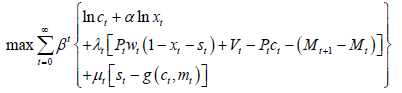On the one hand, as before, the FOCs give the intratemporal condition, i.e. the MRS between consumption and leisure: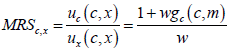This result shows that the MRS between consumption and leisure is equal to the price ratio between them. The numerator represents the total exchange cost of consumption, i.e. the price of consumption plus the value of shopping time.

On the other hand, we can also get the intertemporal condition related to the real money balance:

-wgm (c,m)=R

The left hand side is the marginal benefit of real money, i.e. the real value of time reduced by holding a marginal amount of mt. The right hand side is the marginal cost of real money, i.e. the opportunity cost of mt. This marginal condition shows that the marginal benefit of real money should be equal to the marginal cost it.

For example, Gavin and Kydland assume that shopping time function in their model is: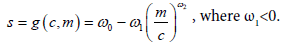This gives a special case of the benchmark model and the resulting money demand is: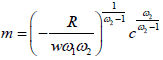Another example is Prescott, who introduces credit in a continuous version. Thus, goods can be purchased by either cash or credit. Assume there is a continuum of shop from 0 to 1. There is a marginal shop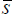where consumer changes the method of purchase. This separates the cash good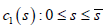and the credit good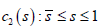. The shopping time for each unit of credit good in store s is denoted by τ(s). By contrast, there is no shopping time for cash good but there is an opportunity cost R. The consumer’s problem becomes: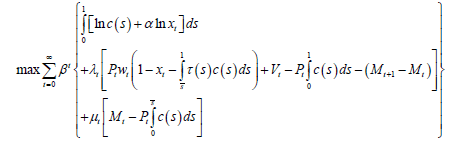Like before, play around with the FOCs in steady state to get the MRS between cash good, credit good and leisure.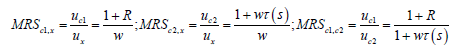Note that the first result does not depend on the marginal store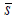. Hence, the result is consistent with Lucas, Cash-in-Advance model and Cash-Credit model. The second result is consistent with the benchmark shopping time model. The numerator represents the total shopping time when consumer uses credit to purchase goods. The last result shows that the MRS between cash good and credit good is equal to the price ratio between them.

The final solution for these control variables can be found by combining the marginal conditions with the budget constraints. Qualitatively, the marginal store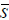can be determined as follows (Graph 3).

Graph 3: Determination of marginal store.

Transaction cost model: Transaction cost is another approach to modelling the role of money in economy, developed by Barsil, Coleman, Schmit, Grobe and Uribe. In these models, there is a transaction cost st when consumer purchases goods, so the total cost of goods is Pt (1+st). Compared to shopping time model where money can reduce the time used in shopping, transaction cost model instead assumes that money can reduce the transaction cost. The feature in common is that money can influence the use of some real resources in economy.

For example, Kimbrough assumes that consumer maximizes: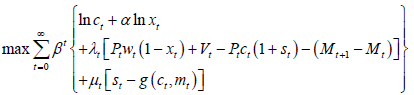As before, the MRS between consumption and leisure can be derived: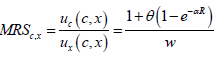This result is consistent with the previous models. The only difference is that the total cost of consumption, represented by the numerator, is equal to the price plus the marginal transaction cost θ (1-e-αR).

The money demand can also be derived based on the FOCs: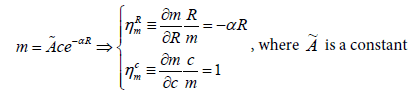Obviously, Cagan (1956) money demand function is a special case of this general form, with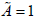and b=α.

Banking approach model: In reality, banking sector provides credit service for consumers to substitute money in transactions. In a general equilibrium model, consumer chooses to buy consumption goods either by money or by credit and allocates time between leisure, good production and credit production.

The benchmark model of this strand assumes that good sector has a simple production function, which only depends on the labour input in good sector: yt=AGt(1-xt-1Qt). The banking sector has a credit production function, which depends on the labour input and deposit input in banking sector: qt AQt lγQt dt1-γ. The proportion of goods purchased by cash is at, so the proportion of goods purchased by credit is 1- at. The government only issue new money supply (vt) each period and transfer it back to the agents. The economy is competitive in all markets. Thus, the centralized equilibrium is equivalent to the decentralized competitive equilibrium. The representative agent’s problem is now: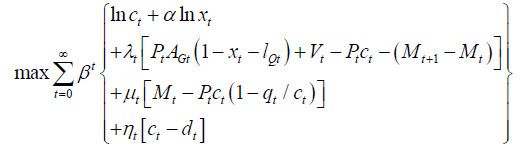There are three constraints. The first is the budget constraint, the second is the CIA constraint for consumption purchased by cash, and the third means the agent’s total consumption should be equal to its total deposit. In some sense, the last is actually a Cash-Credit-In- Advance constraint: agent cannot consume more than his total asset. Though complicated, the essence of this problem is still the same as previous models. We can derive the FOCs and the MRS between consumption and leisure as before: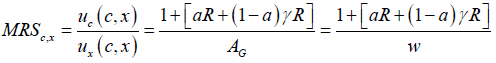The last equation holds if the problem is decentralized and the explicit wage in steady state is just equal to AG. The term [aR+ (1-a) γR] in the numerator represents the weighted average cost of consumption. R is the cost of using money, while γR is the cost of using credit. Hence, the MRS between consumption and leisure is again the price ratio between them.

If we extend the benchmark model by including capital as one of the input factors in banking sectors, then the model will be more general: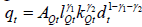.

The representative agent’s problem now becomes: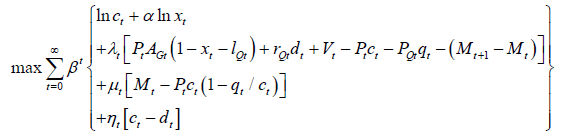Here, the agent receives the interest payment rQtdt as part of its income. Meanwhile, the banking sector now charges PQt for its credit service, rather than for free as in the benchmark model. This charge might come from the interest differential between loan and deposit.

The marginal condition between consumption and leisure (MRSc,x) does not change. However, there is another marginal condition between money and credit, i.e. the marginal cost of credit PQt/Pt should be equal to the marginal cost of money Rt.

Theoretically, the banking approach model is so general that it could include almost all the money demand models as its special case. Firstly, Lucas, CIA model is just a special case when there is no credit, i.e. at=1. In this case, MRS between consumption and leisure is the same as that in CIA model. Secondly, Lucas and Stokey, Cash- Credit model is just a special case when there is no cost of using credit. Thirdly, Shopping Time model can be regarded as a special case where shopping time function st is just equal to the labour input lQt in banking sector. Lastly, the Transaction Cost model is also a special case where the transaction cost function st is equal to the weighted average cost of consumption.

The development in banking sector is prominent in the latest decade before the financial crisis. The productivity of banking sector plays a significant role in determining money demand. The banking approach model is closer to the reality and must provide a better explanation to the real world. Hence, my theoretical model is to be based on this latest development in literature.

#### Empirical Literature Review

Based on the partial equilibrium models, there are enormous empirical studies on estimating the money demand in developed countries. However, empirical studies on that in the developing countries are few. It is because most models are developed in steady state or balanced growth path, which does not apply to transitional path for developing countries.

Estimation of partial equilibrium models

Lucas studies the US money demand and finds that the constant elasticity model does quite well until inflation rose significantly in the US with its peak in early 1980. Then the Cagan constant semi-interest elasticity model does better when there is high variation in the inflation rate. In international panel settings, there tends to be this kind of variability of inflation that is better suited to the Cagan model.

During the US banking deregulation in the 1980’s, many interest bearing instruments became available for consumers, allowing more facile substitution away from money to avoid inflation tax. Many empirical studies found that a stable money demand did not exist, as reported by Friedman and Kuttner, which is referred to as the “missing money” puzzle. This gave rise to the idea that money supply rules could not be used to direct central bank monetary policy because money demand was “unstable”. Hence, it could be said that this laid the foundation for the profession’s broad turning away from money supply rules and back towards interest rate rules as the basis for articulating monetary policy.

An approach to explaining this puzzle is provided by Barnett. He reasoned that a stable money demand function could exist if the noninterest bearing parts of all the different monetary aggregates were taken into account. The composite monetary aggregate that does not yield interest is most like money (Appendix).

Another approach to explaining this puzzle goes back to Friedman to include other factors, such as the prices of “complements” and “substitutes” in addition to “own price” of money, i.e. nominal interest rate R:

M=P.L (R,y, prices of complements and substitutes)

For example, real wage and productivity of banking sector are both associated with the cost of banking and so can enter the money demand function as part of the price of substitutes. Panel data studies can avoid the problem of estimating the unavailable substitute prices by using fixed factors that pick up the effects of abrupt law changes across nations, as in Mark and Sul.

Estimation of general equilibrium models

Gillman and Kejak  formulate a comprehensively nesting general equilibrium model based on banking approach model. It analyzes different classes of endogenous growth models with different usages of physical and human capital, and with different exchange technologies. Theoretically, the banking approach model is the general form of almost all discussed models. Empirically, the banking approach model is also able to characterize the modern world better, since it is close to the workings of the real world. This model works better in four senses.

Firstly, this model also yields a money demand closely comparable to a Cagan model with constant semi-interest elasticity, for which Mark and Sul find recent broad-based cointegration support. Secondly, it successfully generates positive Tobin effects and nonlinear inflationgrowth effects, which are consistent with the real world. Thirdly, this model is also jointly consistent with the Aiyagari, Braun, and Eckstein money and banking findings that the banking sector expands in size in conjunction with the level of the inflation rate. Finally, the employment rate moves in the opposite direction of the inflation rate in the models with human capital. This direction and causality of the employment effect is consistent with evidence in Shadman-Mehta, who finds cointegration of inflation and unemployment for historical UK data, including Phillips original sample period. Moreover, inflation is found to Granger-causes unemployment in the long run.

However, these empirical studies are based on calibration, rather than formal econometric estimation. Typically in RBC analysis, the parameter values of the model are calibrated and not estimated. Kydland and Prescott explain their use of calibration as the result of seeking extraneous information to calibrate the model to the situation of interest, rather than estimating them by traditional econometric methods. They argue that the selection of the parameter values should reflect the specifications of preferences and technology that are used in applied studies, and that they should be those values for which the model’s steady state values are near the average values for the economy over the period being explained. In other words, they want parameter values appropriate for the problem at hand. Hence, the numerical values for the parameters of the model may be obtained from a variety of data sources, e.g. other studies, and not just by traditional econometric estimation methods applied to a single data set. To evaluate a calibrated model, it is common to simulate the variables of the model for some given shocks and then calculate the variance-covariance matrix as well as the autocorrelations and cross correlations. These are then compared with the corresponding second moments of the observed data. Hence, we usually just look at each piece of information rather than a formal way of combining information. By this approach, they bypass many issues related to conventional econometrics. This is indeed a popular way of comparing models, but this methodology cannot give us a formal estimation of money demand function in the econometric sense.

Empirical findings in money demand for China

The studies on the money demand function in China are far behind in both theoretical and empirical aspects. On the one hand, most money demand functions in these empirical studies are developed from partial equilibrium models. The theoretical foundation is weak and obsolete. Recent development in theoretical literature in this area is not yet applied to money demand function study in China. On the other hand, the econometric techniques used are not accurate or even reliable. New empirical methodology is needed to advance the study. Here are some empirical findings based on different methodologies.

Based on quantity theory, Chow  shows that the income elasticity of real M0 demand is 1.16-1.35. Feltenstein and Farhadian find that the income elasticity for real M2 balances in China to be 1.37. Blejer et al. find it to be 1.53. Huang  estimates the income elasticity for nominal M2 is 2.12. Hafer and Kutan  test the existence of a long run nominal money demand function by using cointegration method. Their conclusion for income elasticity for M2 is 1.33. Chen  finds that the income elasticities of the real money demands for M0 and M2 are around 1.4-1.5 and 1.8-1.9.

In contrast to the conventional linear money demand function in the previous findings, there are some studies on nonlinearity of money demand function. Early contribution to this methodology was Sarel, which suggests inflation has a strongly negative impact on economic growth when it rose above 8%. More recent findings by Khan and Senhadji suggest that the threshold level of inflation differs between industrial countries (1%-3%) and developing countries (11%-12%). Austin et al.  apply Terasvirta’s procedure to test the linear money demand function against a smooth transition regression nonlinear alternative. It suggests that there is a critical threshold figure for inflation affecting real money demand in China, at about 5%.

#### Methodology

The methodology of this paper is based on the latest development in literature discussed above. The idea is to incorporate the latest progress in both theoretical and empirical methodologies. It is NOT a simple application of existing method in money demand in China, but a novel innovation to combine the state-of-the-art advances to explain the biggest and most complicated emerging economy.

Theoretical methodology

In the light of the Theoretical Literature Review section, the latest development in theoretical monetary economics lies in banking approach general equilibrium model. As a result, the theoretical model of this paper is based on this latest methodology of modeling. In contrast with the other popular partial equilibrium models, the banking approach general equilibrium model has a theoretical advantage.

Like other general equilibrium models, this banking approach has solid micro foundation. It starts with a representative agent model rather than a system of equations in reduced form. This is the theoretical tendency in macroeconomic models after Lucas Critique, which contends that it is naïve to try to predict the effects of a change in economic policy entirely on the basis of relationships observed in historical data, especially highly aggregated historical data. Because the parameters of those models were not structural, i.e. not policyinvariant, they would necessarily change whenever policy, i.e. the rules of the game, was changed. Policy conclusions based on those models would therefore potentially be misleading. This argument called into question the prevailing large-scale econometric models that lacked foundations in dynamic economic theory. The Lucas critique suggests that if we want to predict the effect of a policy experiment, we should model the “deep parameters” (relating to preferences, technology and resource constraints) that govern individual behavior. We can then predict what individuals will do taking into account the change in policy, and then aggregate the individual decisions to calculate the macroeconomic effects of the policy change. The banking approach model does have this feature.

Moreover, as the second advantage of using banking approach model, compared to other general equilibrium models, banking approach model is comprehensive and flexible. It is proven above that almost all the other models can be regarded as a special case of the banking approach model. That is why Gillman and Kejak find it supported in empirics by calibration.

Empirical methodology

The econometric model, developed from the banking approach, will be estimated together with the conventional partial equilibrium model. This will help compare and appraise the new model with the standard model. On the other hand, the latest advances in time series econometrics are utilized to conduct the inferences.

Firstly, before the two models are estimated, I first test whether all the variables involved in the models are I (1) processes. This step is the basis for further analysis. To improve the power of the tests, both Augmented Dickey-Fuller (ADF) procedure and Kwiatkowski-Phillips- Schmidt-Shin (KPSS) procedure are used.

Secondly, there are also two approaches to test cointegration. The first is based on the residuals from single equation model, i.e. Engle and Granger Approach. If the error term in the model turns out to be stationary while some of the variables in the equation are nonstationary, then these non-stationary variables must be cointegrated with each other. The second is based on the coefficients from multiple equation model or VAR, i.e. Johansen Approach. The single equation can be expressed in a VAR form by defining a new vector containing all the variables. The cointegration test is then based on the coefficient of the rewritten form of this VAR. Both results are reported, but Johansen approach is more reliable due to its theoretical advantage and practical flexibility.

Thirdly, regarding estimation, in addition to the direct regression approach, the second methodology employed is a popular cointegration technique, Error Correction Model (ECM), developed by Engle and Granger. A more advanced technique, Vector Error Correction Model (VECM), is also utilized to match the use of VAR. These two later methodologies are respectively based on single equation analysis and multiple equation analysis, corresponding to the two approaches in cointegration test.

Model

The generality of the model here is embodied by three components: (i) RBC component as the framework as the source of business cycle; (ii) human capital component as the momentum of endogenous growth; (iii) cash-in-advance component as one of the exchange technology; and (iv) banking sector component as the channel of affecting monetary economy. The last point is the key feature to include other models as its special cases. Hence, as termed in Gillman and Kejak, this comprehensive model is called "General Monetary Endogenous Growth Model". Since we adopt the neoclassical doctrine that the market is competitive and prices are flexible to clear the market, it is equivalent to use representative agent model rather than a decentralized model. The only difference is that all the prices are now implicit.

The model has three sectors that use both physical capital and human capital augmented labour: good sector, banking sector and human capital sector.

The representative agent’s problem

The representative agent maximizes the life-time discounted utility, with an instantaneous utility function:

u (ct,xt)=lnct+αlnxt

The agent’s first constraint is resource constraint. There are two sources of income: (i) output produced by the good sector and (ii) lumpsum transfer form government. There are four ways of expenditures: (i) consumption purchase ct; (ii) physical investment kt+1- kt (1-δk); (iii) financial investment (bond) Bt+1 - Bt (1+Rt); and (iv) money holding Mt+1 -Mt.

PtAG (sGt kt)θ(lGt ht)1-θ+Vt=Ptct+Pt [kt+1-kt(1-δk)]+[Bt+1-Bt(1+Rt)]+(Mt+1-Mt)

As mentioned above, in the competitive market, this is equivalent to the decentralized model. In the decentralized model, the consumer has three sources of income: (i) labour income from good sector (human capital augmented); (ii) capital income from good sector; (iii) the profit from the good sector, which is zero in competitive market; and (iv) a lump-sum transfer form government. Hence, the resource constraint can also be equivalently expressed as:

PtwtlGtht+PtrtsGtkt+Vt=Ptct+Pt [kt+1-kt(1-δk)]+[Bt+1-Bt(1+Rt)]+(Mt+1-Mt)

The agent’s second constraint is exchange constraint, i.e. to allocate his consumption purchase between money and credit. The real credit qt is the residual amount of real consumption ct not purchased by money. at is the fraction of purchase by money.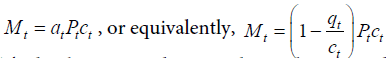The banking sector has a credit production technology: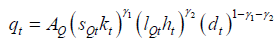There are three input factors in this technology: physical capital sQtkt, human capital augmented labour lQtht, and bank deposit dt. This technology is constant returns to scale. In equilibrium, the total consumption must be equal to total deposit, i.e. dt=ct. Combine these results to obtain the exchange constraint: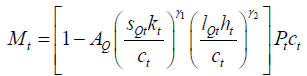The agent’s third constraint is human capital constraint, describing the law of motion for the human capital sector.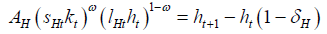There are another two endowment constraints, which can be substituted into the three constraints for simplicity. The first endowment constraint is to allocate his time endowment between leisure xt, labour in good sector lGt, labour in human capital sector lHt and banking sector lQt:xt+lGt+lQt+lHt=1. The second endowment constraint is to allocate his capital endowment between good sector sGt, banking sector sQt and human capital sector: sGt+ sQt+sHt=1. These two constraints can be used to reduce the number of control variables. In this case, lHt and sHt are substituted out.

Hence, the representative agent’s problem can be formulized as: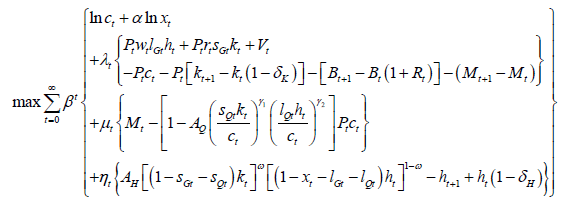First order conditions

The FOCs of this maximization problem can be obtained by taking derivatives with respect to the ten control variables {ct,xt,lGt,lQt,Mt+1,Bt+1, kt+1,ht+1,sGt,sQt} as well as the Lagrangian multipliers {λttt}, which are just the three constraints.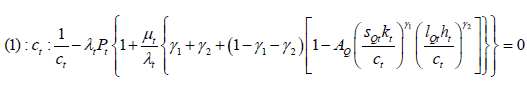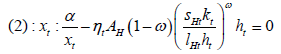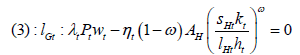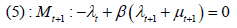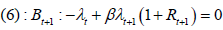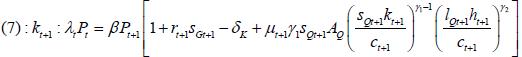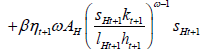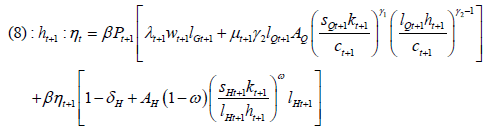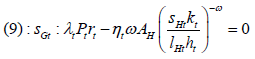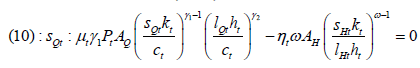In equilibrium, the government supplies nominal money through the lump-sum transfer at a steady rate, i.e. Mt+1-Mt=Vt. Moreover, in equilibrium, the government cannot let its bond grow because that will violate the transversality condition, i.e. Bt+1=Bt (1+Rt). Based on these two equilibrium conditions, the three constraints (which are also the rest three FOCs) now become: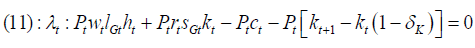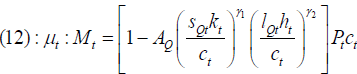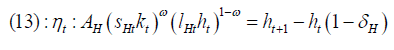Marginal conditions

Similar to the procedures in the Theoretical Literature Review, we can utilize these FOCs to obtain intertemporal and intratemporal conditions.

Intratemporal condition: The intratemporal condition is obtained by combing the FOCs (1) and (2) with respect to consumption and leisure. The result is a marginal condition within each period, i.e. the MRS between ct and, xt which describes the trade-off between the two components in the utility.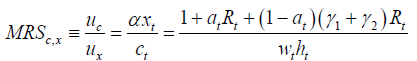This result is consistent with the previous conclusion that the margin is equal to the price ratio. The total price of consumption is equal to the shadow price of consumption (1) plus the exchange cost of the weighted average of using money (atRt) and using credit (atRt+(1-at)(γ12)Rt).

Intertemporal condition: After some derivations, we can obtain two important intertemporal conditions. The first one is Euler’s equation, which describes the balanced growth path of real aggregates such as output, consumption, physical capital and human capital.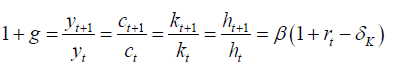This result shows that the growth rate is not affected by nominal variables. It is because the nominal variables cannot affect the physical or human capital investments, since capital does not have to be purchased by money. It is almost consistent with the reality in that people tend to borrow money from bank and invest in their machine or education by credit. However, it is not precisely the case because people do have to provide mortgage or prepay a fraction of the total amount. The more general model assumes that a proportion a2 of investment is also paid by money. In this case, the nominal variable Rt will enter the balanced growth path with a negative effect.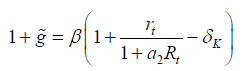The second intertemporal condition is Fisher’s equation, which can be derived from FOC (6) with respect to government bond Bt+1.

1+Rt=(1+πt) (1+rtk)

This result shows that the nominal rate of return is equal to the inflation rate times the real rate of return to capital. Basically, it is a simple no-arbitrage condition in the efficient market, i.e. the return in financial market by investing in financial capital should be equal to that in good market by investing in physical capital.

Money demand function

In principle, all the ten control variables can be solved, including money demand, in terms of only state variables and constant parameters. In this paper, I only focus on the money demand Mt. Make use of the exchange constraint and combine it with FOCs to obtain the real money demand function in terms of ct: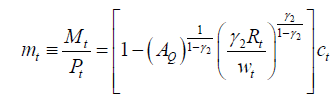Rewrite this equation by dividing both hand sides by real output yt: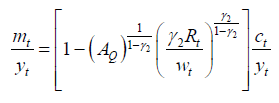The solution for ct/yt is a constant, which can be shown by using the marginal conditions. This result is consistent with the permanent income hypothesis in the long run. Hence, the money demand function reduces to: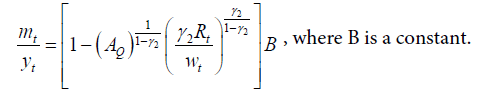This result shows that the money demand per output (i.e. the inverse velocity) depends negatively on the nominal interest rate Rt, positively on the real wage Wt, and negatively on the level of productivity in the banking sector AQ. Indeed, financial innovation has been considered as a factor of money demand in the literature. The inclusion of the banking sector as a time series variable is advocated by Gillman and Otto , and their model is a simplified version of this comprehensive framework.

To make the money demand function a tractable form, we can use the approximation rule: ln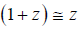, if z is small. Define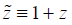, then the rule can be rewritten as ln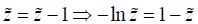. In this case, the second term in the square bracket is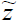, so the equation is transformed as: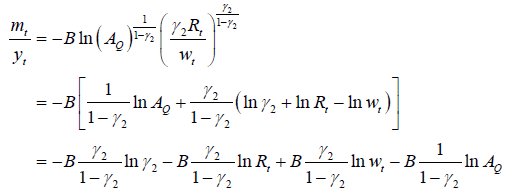Further, the equilibrium money demand approximation can be expressed as an econometric model for estimation: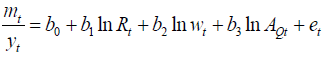The left hand side is the inverse velocity equal to the real money demand (mt) divided by the real GDP output (yt). The right hand side consists of a constant term, nominal interest rate (Rt), real wage level (Wt) and the productivity of banking sector (AQt). et is assumed to be a stationary error term, which reflects dynamic adjustment, measurement errors and omitted variables.

However, as pointed out by Chen , the official interest rate (Rt) in China is not a good measure for the opportunity cost of holding money since they are often arbitrarily set by the government and they change only very infrequently. It is convenient to use inflation (πt) to measure the opportunity cost of holding money in the light of Fisher’s equation, which is derived earlier in the intertemporal condition. Moreover, the productivity of banking sector (AQt) is not available directly. As suggested by Gillman and Otto, the real wage in the banking sector (WQt) is a good proxy measure. Provided factor markets are competitive, changes in the real wage will reflect productivity changes. Lowe also supports this use in Australian case.

The revised econometric model is denoted as [Model 1]: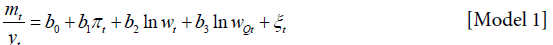[Model 1] is significantly different from those in other empirical literature, which share the following form [Model 0] developed from partial equilibrium theory: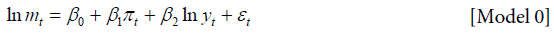On the one hand, the dependent variable in [Model 1] is the level of inverse velocity, while that in [Model 0] is the logarithm of real money demand. On the other hand, there are two more independent variables. These differences then result in different elasticities of money demand.

Firstly, from the partial equilibrium theory, the coefficient β1 of [Model 0] is expected to be negative, which represents the interest elasticity of money demand. In contrast, based on the banking approach specification [Model 1], the interest elasticity is derived as coefficient b1 multiplied by the velocity. Thus, the coefficient b1 is also expected to be negative, but it has a Cagan type feature that the interest elasticity varies across different levels of velocity.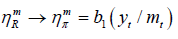Secondly, the coefficient β2 in [Model 0] is the income elasticity of money demand, which is expected to be positive and close to 1, but the actual value is yet to be estimated. Other empirical findings support the hypothesis that the income elasticity is close to 1. To make use of this information in [Model 1], the income elasticity is restricted to be equal to 1 by structure. This restriction, if correctly imposed, is supposed to improve the estimation.

Data

All the data are annual from 1979 to 2007, after the reform in 1978. The national account variables, such as GDP, price index and wages, are taken from the National Bureau of Statistics (NBS) of China. The money aggregates, M0 and M1, are taken from the central bank, i.e. the People’s Bank of China (PBOC).

In particular, for the price data, I follow Chow to use the retail price index (RPI), since the results are not affected by using different price indices. Inflation, real GDP and real wages are calculated by RPI. Real GDP can also be calculated by deflator, but the result shows again there is no significant difference. Here, to be consistent, all the real variables are based on RPI. For the money aggregates, M0 only consists of currency in circulation, while M1 also include the deposits people can withdraw anytime without additional transaction costs by debit card or over the counter. Both M0 and M1 are used for robustness analysis of the model. The real money balances are also calculated by RPI.

One vital problem with data is that the wage in banking sector (WQt) is only available after 1999. There are two ways to complete the data back to 1979. The first way is to extrapolate the data back to 1979. However, the period to be extrapolated is too long (20 years), compared to the data (10 years). In addition, there were dramatic changes during those 20 years, which made the extrapolation unreliable. Alternatively, a variable closely related to WQt without omitted data can be employed to proxy WQt. For this purpose, wage in states-owned sector is appropriate, since banking sector is a part of states-owned sector in China. Moreover, in China, the wage in states-owned sector is approximately similar, especially in the early years. The wage was planned and distributed by the central government independent of local government. Hence, the two should be close not only in relative change, but also in level. As predicted, the correlation between bank sector and states-owned sector between 1999~2007 turns out to be 0.964, which is very close to perfect correlation. Based on this argument and result, we can use wage of states-owned sector, the data of which is available from 1979 to 2007, to proxy the wage of banking sector.

#### Result

Stationary test

To estimate the econometric models, stationary tests are essential to provide direction for estimation technique. The Graphs 4 and 5 shows the time paths of the variables in both models. It is likely that log real money (both lnm0t and lnmlt), log output (lnyt), log real wage (lnWt) and log real wage in banking sector (lnWQt) are nonstationary, probably with intercepts and trends. By contrast, inflation (πt) and inverse velocity of real money (both m0t/yt and mlt/yt) seem to be stationary. As a result, strict statistical tests are needed. The results of ADF and KPSS tests for all the variables involved in both models are reported in Table 1. The two sets of results are generally consistent.

t-statistic p-value LM-statistic significance level
lnmot -2.637258 0.2681 0.099165 >10%
lnmlt -1.484428 0.8100 0.152703 <5%
lnyt -2.016794 0.5664 0.157137 <5%
πt -1.748625 0.0763 0.221604 >10%
mot/yt -0.375646 0.9004 0.555889 <5%
mlt/yt -1.052249 0.7199 0.486165 <5%
lnwt -3.113513 0.1241 0.077496 >10%
lnwQt -2.363598 0.3886 0.091048 >10%

Table 1: Summary of Stationary Test.

According to ADF test, the null hypothesis is that the variable in question has a unit root. At the one extreme, the p-value is greater than 70% for log real M1 (lnmlt), inverse velocity of real M1 (mlt/yt) and real M0(m0t/yt). That suggests a strong nonstationarity of these variables. At the other extreme, inflation (πt) is quite close to stationary with a low p-value 7.63%, which means that the unit root hypothesis for πt is quite weak. However, it is still nonstationary by convention. Other variables, such as log real output (lnyt), log real M0 (lnm0t), log real wage (lnwt) and log real wage in banking sector (lnwQt) have p-values around 30%.

By contrast, according to the KPSS test, the null hypothesis is that the variable in question is stationary. The results are generally consistent with ADF test. The significance levels of lnmlt, lnyt, lnyt, m0t/ yt, and mlt/yt are less than 5% but higher than 1%. This finding is exactly the same as in ADF test. In addition, the significance levels of other variables revolve 10%, representing a weak stationarity feature.

Combining the two sets of results, we can conclude that all the variables in the two models are I (1) nonstationary processes, though inflation is quite close to stationary. This finding suggests that there must be cointegration relationship between them, if the error terms, et and εt are both stationary processes.

Cointegration test

To test the cointegration relationship between the variables in the models, there are two approaches employed. The first one is based on the residuals of the single equation models, i.e. Engle and Granger Approach. The second one is based on the coefficients from multiple equation model or VAR, i.e. Johansen Approach.

Engle and Granger approach: This approach is carried out by first estimating the models directly and then testing the stationarity of resulting residuals. To see the robustness of the models as shown in Table 2, both M0 and M1 are used for money demand values. The regression results and test statistics are presented in Tables 3 and 4 for [Model 0], while in Tables 5 and 6 are for [Model 1]. The ARMA structures of the models are determined based on the correlograms and Q-statistics of the regression residuals. These cointegration test results are summarized below:

Money Aggregate Model ADF t-statistic P-value
M0 [Model 0] -3.381646 0.0016
[Model 1] -2.945005 0.0049
M1 [Model 0] -3.055022 0.0037
[Model 1] -3.110752 0.0032

Table 2: Cointegration test results.

Variable Coefficient Std. Error t-Statistic Prob.
pt -0.18425 0.452024 -0.4076 0.6875
ln yt 0.615753 0.084706 7.269273 0
C -0.11669 0.637995 -0.1829 0.8566
AR(1) 1.244522 0.202781 6.13728 0
AR(2) -0.52063 0.18294 -2.84593 0.0094
R-squared 0.970147  Mean dependent var 4.425448
Adjusted R-squared 0.96472  S.D. dependent var 0.51894
S.E. of regression 0.097473  Akaike info criterion -1.65291
Sum squared resid 0.209021  Schwarz criterion -1.41294
Log likelihood 27.31427  F-statistic 178.7381
Durbin-Watson stat 1.972748  Prob(F-statistic) 0
Inverted AR Roots  .62+.37i  .62-.37i
ADF test for the residuals from the regression above:
t-Statistic  Prob.*
Augmented Dickey-Fuller test statistic -3.38165 0.0016
Test critical values: 1% level -2.66485
5% level -1.95568
10% level -1.60879

Table 3: Engle and Granger Test in [Model 0] Using M0.

Variable Coefficient Std. Error t-Statistic Prob.
pt -0.991309 0.393024 -2.52226 0.019
ln yt 1.754043 0.561339 3.124747 0.0048
C -27.0975 144.263 -0.187834 0.8527
AR(1) 0.995289 0.032448 30.67289 0
MA(1) 0.473124 0.191006 2.47701 0.021
R-squared 0.9808  Mean dependent var 5.630857
Adjusted R-squared 0.977461  S.D. dependent var 0.650569
S.E. of regression 0.09767  Akaike info criterion -1.654016
Sum squared resid 0.219406  Schwarz criterion -1.416122
Log likelihood 28.15622  F-statistic 293.7309
Durbin-Watson stat 1.797574  Prob(F-statistic) 0
Inverted AR Roots  1.00
Inverted MA Roots  -.47
ADF test for the residuals from the regression above:
t-Statistic  Prob.*
Augmented Dickey-Fuller test statistic -3.05502  0.0037
Test critical values: 1% level -2.65692
5% level -1.95441
10% level -1.60933

*MacKinnon (1996) one-sided p-values.

Table 4: Engle and Granger Test in [Model 0] Using M1.

Variable Coefficient Std. Error t-Statistic Prob.
πt -0.044191 0.061686 -0.716383 0.4810
ln wt -0.082886 0.070362 -1.177993 0.2508
ln wQt 0.045367 0.059300 0.765034 0.4520
C 0.232052 0.054670 4.244626 0.0003
AR(1) 0.717191 0.141872 5.055182 0.0000
R-squared 0.903852  Mean dependent var 0.055862
Adjusted R-squared 0.887131  S.D. dependent var 0.019968
S.E. of regression 0.006708  Akaike info criterion -7.010514
Sum squared resid 0.001035  Schwarz criterion -6.772620
Log likelihood 103.1472  F-statistic 54.05370
Durbin-Watson stat 1.558085  Prob(F-statistic) 0.000000
Inverted AR Roots   .72
ADF test for the residuals from the regression above:
t-Statistic  Prob.*
Augmented Dickey-Fuller test statistic -2.945005  0.0049
Test critical values: -2.656915 -2.65692
-1.954414 -1.95441
-1.609329 -1.60933

Table 5: Engle and Granger Test in [Model 1] Using M0.

Variable Coefficient Std. Error t-Statistic Prob.
πt -0.625123 0.160950 -3.883960 0.0008
ln wt -0.680319 0.210037 -3.239041 0.0036
ln wQt 0.513908 0.182163 2.821146 0.0097
C 0.874456 0.114997 7.604139 0.0000
AR(1) 0.542485 0.212430 2.553709 0.0178
R-squared 0.900704  Mean dependent var 0.191541
Adjusted R-squared 0.883435  S.D. dependent var 0.065433
S.E. of regression 0.022340  Akaike info criterion -4.604459
Sum squared resid 0.011479  Schwarz criterion -4.366565
Log likelihood 69.46242  F-statistic 52.15761
Durbin-Watson stat 1.691369  Prob(F-statistic) 0.000000
Inverted AR Roots  .54
ADF test for the residuals from the regression above:
t-Statistic  Prob.*
Augmented Dickey-Fuller test statistic -3.110752  0.0032
Test critical values: 1% level -2.65692
5% level -1.95441
10% level -1.60933

Table 6: Engle and Granger Test in [Model 1] Using M1.

As shown in the summary table, the probability of accepting the null hypothesis that the residual process has a unit root is quite small, all less than 0.005 or 0.5%. We can then conclude with high confidence that there exist cointegration relationships and the coefficients are the cointegration vector for each model. In [Model 0], the cointegration vector for [lnmt, πt, lnyt] is [1,0.18,-0.62] if M0 is used or [1, 0.99, -1.75] if M1 is used. Similarly, in [Model 1], the cointegration vector for [mt/ytπt, lnwt, lnwQt] is [1, 0.04, 0.08, -0.04] if M0 is used or [1, 0.63, 0.68, -0.51] if M1 is used.

Johansen approach: Engle and Granger approach is straightforward and easy to implement, but the drawback is that it pre-assumes the dependent variables. In contrast, Johansen approach is based on VAR model, which does not impose any restrictions on which variable is dependent. [Model 0] can be rewritten in a form of structural VAR: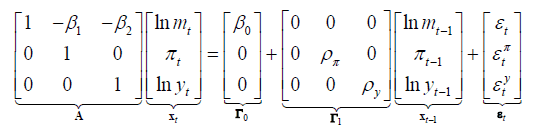Assume that the coefficient matrix A is invertible, then the reduced VAR form is: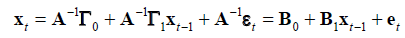The VAR model can then be rewritten in the VECM form: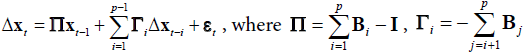All the terms except Xt are I(0) by differencing or by nature. Hence, the Johansen test of cointegration is based on the coefficient Π of the VECM.

(i) If the rank of the coefficient matrix r=rank (Π) is equal to the dimension of k=dim (xt), then all of the components in xt should be I(0). It contradicts the results of stationarity test that they are actually I(1).

(ii) If the rank r=0, then Π=0, which implies that there is no cointegration relationship between the components in xt.

(iii) If the rank 0 < r < k, then there are r cointegrating vectors.

The null hypothesis and alternative hypothesis are:

Hr0: λr > 0,r+1=0 V.S. Hr1: λr+1 > 0, r=0,1,…., k-1

The result of Johansen tests of cointegration for [Model 0] are presented in Table 7 for M0 and M1 respectively. It is shown by both trace test and maximum eigenvalue test that there is at most 1 cointegrating equation at the 0.05 significance level. This finding is consistent with the assumption under Engle and Granger approach.

Unrestricted Cointegration Rank Test (Trace)
Hypothesized   Trace 0.05
No. of CE(s) Eigenvalue Statistic Critical Value Prob.**
None *  0.797957  69.07530  42.91525  0.0000
At most 1  0.434264  24.29565  25.87211  0.0776
At most 2  0.257754  8.346093  12.51798  0.2247
Unrestricted Cointegration Rank Test (Maximum Eigenvalue)
Hypothesized   Max-Eigen 0.05
No. of CE(s) Eigenvalue Statistic Critical Value Prob.**
None *  0.797957  44.77966  25.82321  0.0001
At most 1  0.434264  15.94955  19.38704  0.1474
At most 2  0.257754  8.346093  12.51798  0.2247
Johansen cointegration test between ln t m , t π and ln t y for M1 in [Model 0]:
Unrestricted Cointegration Rank Test (Trace)
Hypothesized   Trace 0.05
No. of CE(s) Eigenvalue Statistic Critical Value Prob.**
None *  0.836306  68.96871  42.91525  0.0000
At most 1  0.341216  18.29558  25.87211  0.3244
At most 2  0.210262  6.609509  12.51798  0.3870
Unrestricted Cointegration Rank Test (Maximum Eigenvalue)
Hypothesized   Max-Eigen 0.05
No. of CE(s) Eigenvalue Statistic Critical Value Prob.**
None *  0.836306  50.67313  25.82321  0.0000
At most 1  0.341216  11.68607  19.38704  0.4449
At most 2  0.210262  6.609509  12.51798  0.3870

Table 7: Johansen Test in [Model 0] Johansen cointegration test between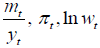and ln wQt for M0 in [Model 1].

Similarly, for [Model 1], the procedures are exactly the same except for the components contained for xt and the coefficient matrices.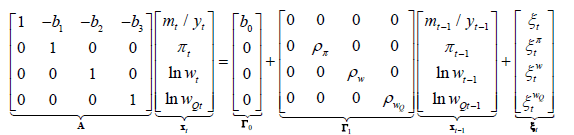Assume that the coefficient matrix A is invertible, then the reduced VAR form is: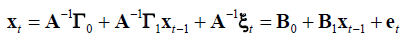The VAR model can then be rewritten in the Vector Error Correction (VEC) form: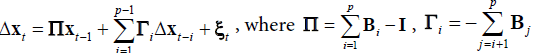The result of Johansen tests of cointegration for [Model 1] are presented in Table 8 for M0 and M1 respectively. There is a contradiction between trace test and maximum eigenvalue test if M0 is used. The trace test results in at most 2 cointegrating equations while maximum eigenvalue test supports at most 1 cointegrating equation at the 0.05 significance level. The results for M1 are both at most 1 cointegrating equation.

Unrestricted Cointegration Rank Test (Trace)
Hypothesized   Trace 0.05
No. of CE(s) Eigenvalue Statistic Critical Value Prob.**
None *  0.727076  81.22330  63.87610  0.0009
At most 1  0.547380  44.86359  42.91525  0.0315
At most 2 0.471034 22.66790 25.87211 0.1191
At most 3  0.158641  4.836637  12.51798  0.6199
Unrestricted Cointegration Rank Test (Maximum Eigenvalue)
Hypothesized   Max-Eigen 0.05
No. of CE(s) Eigenvalue Statistic Critical Value Prob.**
None*  0.727076  36.35971  32.11832  0.0142
At most 1 0.547380 22.19569 25.82321 0.1403
At most 2  0.471034  17.83126  19.38704  0.0829
At most 3  0.158641  4.836637  12.51798  0.6199
Johansen cointegration test between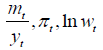and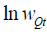for M1 in
[Model 1]:
Unrestricted Cointegration Rank Test (Trace)
Hypothesized   Trace 0.05
No. of CE(s) Eigenvalue Statistic Critical Value Prob.**
None *  0.682211  57.58211  47.85613  0.0047
At most 1 0.537540 26.63019 29.79707 0.1110
At most 2  0.173375  5.807933  15.49471  0.7181
At most 3  0.024402  0.667017  3.841466  0.4141
Unrestricted Cointegration Rank Test (Maximum Eigenvalue)
Hypothesized   Max-Eigen 0.05
No. of CE(s) Eigenvalue Statistic Critical Value Prob.**
None *  0.682211  30.95192  27.58434  0.0178
At most 1 0.537540 20.82225 21.13162 0.0552
At most 2  0.173375  5.140917  14.26460  0.7239
At most 3  0.024402  0.667017  3.841466  0.4141

* denotes rejection of the hypothesis at the 0.05 level
**MacKinnon-Haug-Michelis (1999) p-values

Table 8: Johansen Test in [Model 1] ECM estimation result of [Model 0] for M0 with an AR(2) error term: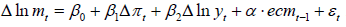Based on the two approaches, we can now accept with high confidence that there is only 1 cointegrating equation in both models under both measures of money supply. This conclusion lays the foundation for estimation using VECM representation of the single equation models.

Estimation

Direct approach: The simplest way of estimating the two models is to run direct regression with a specified structure for the error term. Actually, the regressions to run are exactly the same as those for cointegration tests by Engle and Granger Approach. The estimated coefficients as shown in Table 9 are just the results by the direct regression approach.

Variable Coefficient Std. Error t-Statistic Prob.
Dpt 0.105070 0.401988 0.261376 0.7968
D ln yt 1.921966 0.536143 3.584799 0.0021
ecmt-1 -0.409000 0.194171 -2.106391 0.0495
C -0.137279 0.067562 -2.031915 0.0572
AR(1) 0.937869 0.214214 4.378181 0.0004
AR(2) -0.483883 0.215230 -2.248218 0.0373
R-squared 0.575496  Mean dependent var 0.069408
Adjusted R-squared 0.457578  S.D. dependent var 0.129355
S.E. of regression 0.095269  Akaike info criterion -1.651913
Sum squared resid 0.163370  Schwarz criterion -1.357399
Log likelihood 25.82295  F-statistic 4.880484
Durbin-Watson stat 1.976570  Prob(F-statistic) 0.005372
Inverted AR Roots  .47+.51i  .47-.51i
ECM estimation result of [Model 0] for M1 with an ARMA(1,l) error term: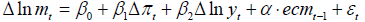Variable Coefficient Std. Error t-Statistic Prob.
Dpt -2.016102 0.158939 -12.68478 0.0000
D ln yt 1.762568 0.140245 12.56777 0.0000
ecmt-1 1.446773 0.080209 18.03763 0.0000
C -0.097062 0.014657 -6.622019 0.0000
AR(1) -0.465317 0.211045 -2.204825 0.0393
AR(2) -0.939344 0.100783 -9.320420 0.0000
R-squared 0.779654  Mean dependent var 0.080208
Adjusted R-squared 0.724567  S.D. dependent var 0.131743
S.E. of regression 0.069141  Akaike info criterion -2.306167
Sum squared resid 0.095609  Schwarz criterion -2.015837
Log likelihood 35.98017  F-statistic 14.15325
Durbin-Watson stat 2.058771  Prob(F-statistic) 0.000005
Inverted AR Roots  -.47
Inverted MA Roots  .94

Table 9: ECM Estimation for [Model 0] ECM estimation result of [Model 1] for M0 with an AR(1) error term: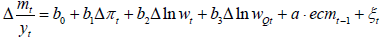For [Model 0] when M0 is used, the error term is justified to have an AR (2) structure. The coefficient of log real income lnyt is highly significant and positive around 0.62, which implies that the income elasticity of money demand ηmy is less than 1. This finding is a bit different from earlier empirical findings that ηmy for M0 is greater than 1, e.g. 1.16-1.35 in Chow and 1.4-1.5 in Chen. As we shall see later, this result might be improved by more advanced estimation approaches.

Moreover, the estimated coefficient of inflation πt, which is supposed to reflect the interest elasticity of money demand ηmy , is negative but not significant. That means the change in opportunity cost of money does not have significant effect on money demand. It can of course be explained by the argument that demand for cash is quite inelastic, because we have to use cash for a certain amount of purchases, especially in China where banking sector has not developed to an advanced level. Hence, even if there is a high opportunity cost, people could not find enough alternatives to cash. Although it has a tendency of being negative, it is still not significantly different from 0 in statistical sense.

For [Model 0] when M1 is used, the error term is specified as a ARMA (1, 1) process. The coefficient of log real income lnyt is highly significant and positive around 1.75, which implies that the income elasticity of money demand ηmy is consistent with previous empirical findings, e.g. 1.8-1.9 in Chen. It implies that the income elasticity of M1 is higher than that of M0. It is probably because people have to substitute their term deposits by cash or current deposits when they demand more transactions. By contrast, demand for M0 is not that sensitive because the transactions made by cash are relatively stable. The increase in demand for transactions mainly lies in those that can be done by both M0 and M1.

On the other hand, there is a very nice result for interest elasticity ηmR, which turns out to be equal to -0.99. It is not significantly different from the theoretical value of -1 by Wald test. This finding supports the MIUF theory that ηmR=-1 and the Baumol type models with constant and negative interest elasticity . This level seems to be a bit high in absolute value, since most empirical findings support a ηmR close to -0.5. Although it provide a reasonable qualitative outcome, the quantitative feature is not perfect. It might be due to the estimation technique, so more advanced approaches are applied to see whether there is any improvement.

For [Model 1] when M0 is used, the error term is justified to have an AR (1) structure. As analyzed earlier, the income elasticity of money is assumed to be 1 by structure of the model. The resulting interest elasticity ηmR is equal to b1 (yt/mt), which will vary with the velocity. The estimate of b1 under M0 is not significantly different from 0, although negative. It gives a similar result as [Model 0] when M0 is used, where the estimate of β1 is also insignificant. The real wage lnwt and banking sector productivity proxy lnwQt are not significant either. However, the theoretical model does improve the plausibility of the result as shown later.

For [Model 1] when M1 is used, the error term is justified to have an AR (1) structure. The estimation result for this specification is more favorable. Firstly, the estimate of b1 is significantly negative around -0.625. Combined with the velocity of money, we can generate the varying ηmR, shown in Graph 6. As we can see, ηmR is extremely high in absolute value (around -5) in the first half of 1990’s. It is because of the high inflation in China during that period. People are more sensitive to inflation and tend to substitute their money holdings. This is in line with the Cagan model, which is mainly suitable for hyperinflation phenomenon. As more and more attention has been drawn to controlling inflation by Chinese authority, the inflation has been more and more stable, and the interest elasticity has converged back to a steady state level around -3.5 since 1998. Secondly, the other terms are now significant such as lnwt and lnwQt. It implies that these factors are essential in explaining money demand. In particular, the real wage has a negative effect (around -0.68) on inverse velocity, or equivalently, it has a positive effect on velocity. Intuitively, the higher wage people earn, the more demand for transactions are induced. By contrast, the banking sector productivity has a positive effect (around 0.51) on inverse velocity, that is to say, the higher productivity of the banking sector, the slower the velocity of money circulation. That makes sense because people could use banking services to substitute the cash transactions, resulting in slower velocity.

Error correction model (ECM) approach: The ECM approach is based on single equation econometric model, proposed by Sargen and formalized by Engle and Granger. To estimate the two models, we have to rewrite them in ECM form, where α and a are adjustment coefficients.

Δ lnmt0 + β1 Δπt + β2 Δlnyt + α.ecmt-1 + εt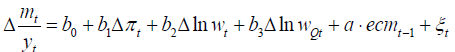The procedure of ECM estimation is as follows:

(i) Run the cointegrating regression of the original explained variable on the explanatory variables in the original model;

(ii) Replace the ECM term in the ECM model by residuals from (i).

The regression results for [Model 0] are presented in Table 10. When M0 is used, the estimate for interest elasticity of money is still insignificant, but the estimate for income elasticity of money is higher (around 1.92) compared to previous result (0.62) by direct approach. This is a big discrepancy in quantity since one is less than 1 while the other is much greater than 1. Arguably, the new result is more reliable because it is similar to other empirical studies and it has plausible explanations. The high interest elasticity is due to various alternatives to cash developed by banking sectors, such as credit card services and debit card services. When M1 is used, the estimate for income elasticity of money is around 1.76, similar to the result (1.75) obtained by direct approach. It implies that the result of M1 is robust to estimation method.

Variable Coefficient Std. Error t-Statistic Prob.
Dpt -0.098820 0.054870 -1.800971 0.0868
D ln wt -0.055594 0.057830 -0.961336 0.3479
D ln wQt 0.123902 0.060345 2.053229 0.0534
ecmt-1 -0.412662 0.256292 -1.610122 0.1230
C -0.009346 0.003921 -2.383342 0.0272
AR(1) 0.614836 0.206408 2.978735 0.0074
R-squared 0.394628  Mean dependent var -0.001572
Adjusted R-squared 0.243285  S.D. dependent var 0.006461
S.E. of regression 0.005620  Akaike info criterion -7.325731
Sum squared resid 0.000632  Schwarz criterion -7.035401
Log likelihood 101.2345  F-statistic 2.607507
Durbin-Watson stat 1.436756  Prob(F-statistic) 0.056801
Inverted AR Roots  .61
ECM estimation result of [Model 1] for M1 with an MA(1) error term: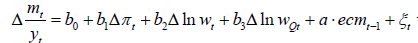Variable Coefficient Std. Error t-Statistic Prob.
Dpt -0.641100 0.174517 -3.673571 0.0014
D ln wt -0.495504 0.195712 -2.531806 0.0194
D ln wQt 0.624849 0.183561 3.404047 0.0027
ecmt-1 -0.657965 0.174394 -3.772872 0.0011
C -0.021985 0.010204 -2.154454 0.0430
MA(1) 0.997478 0.103701 9.618819 0.0000
R-squared 0.537342  Mean dependent var -0.004118
Adjusted R-squared 0.427185  S.D. dependent var 0.023167
S.E. of regression 0.017534  Akaike info criterion -5.056259
Sum squared resid 0.006456  Schwarz criterion -4.768295
Log likelihood 74.25950  F-statistic 4.877976
Durbin-Watson stat 2.357988  Prob(F-statistic) 0.004069
Inverted MA Roots  -1.00

Table 10: ECM Estimation for [Model 1] VECM regression result of [Model 0] for M0 and M1: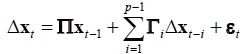When M0 is used.

Similarly, the regression results for [Model 1] are in Table 10. When M0 is used, the income elasticity of money is assumed to be 1 by structure of the model. The resulting interest elasticity ηmR is equal to b1 (yt/mt), which will vary with the velocity. The estimate of b1 under M0 is still insignificant (at 5% level). It gives a similar result as [Model 0] when M0 is used, where the estimate of β1 is also insignificant. The real wage lnwt is still insignificant but banking sector productivity proxy lnwQt is now significant. Hence, the theoretical model does improve the estimation, although little.

When M1 is used, the estimate of b1 is significantly negative around -0.641, which is quite similar to the result (-0.625) obtained under direct method. It again supports the conclusion that the estimate for M1 is robust to estimation methods. Moreover, the other terms are now significant such as lnwt and lnwQt, implying that these factors are essential in money demand function. In particular, the real wage has a negative effect (around -0.50) on inverse velocity, or equivalently, it has a positive effect on velocity. By contrast, the banking sector productivity has a positive effect (around 0.62) on inverse velocity. Compared to the results obtained earlier, they are consistent in both qualitative and quantitative aspects.

Vector error correction model (VECM) approach: Based on the VECM representation above, we can estimate the coefficients in by multiple equation approach. To begin with, the two models are now rewritten as: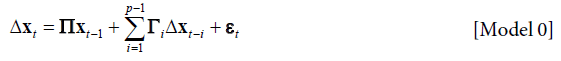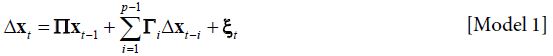The estimation results of the VECM are illustrated in Tables 11 and 12. The coefficients of cointegrating equation are just the cointegrating vector, which can be used as the estimates of elasticities. The results show huge discrepancies with the earlier results. For [Model 0], the income elasticity of money demand ηmy is equal to 1.19 when M0 is used and 0.56 when M1 is used. The estimate of interest elasticity of money demand ηmR is 13.91 and -11.62 respectively, which are quite unlikely to be reliable, because interest elasticity should be negative and around -1. Thus, the new approach does not improve the plausibility of elasticity estimates based on [Model 0]. That is to say, the estimate of the partial equilibrium model is not robust to the estimation method. It might be due to the naïve misspecification of the partial equilibrium model, which omits other important factors like productivity of banking sector. Although the results are worse than those found under other estimation approaches, it reveals the weakness of the model itself in robustness. In other words, it shows support to replace the traditional model by alternative general equilibrium model.

 Vector Error Correction Estimates Sample (adjusted): 1982 2007 Included observations: 26 after adjustments Standard errors in ( ) & t-statistics in [ ] Cointegrating Eq: CointEq1 LNM0(-1) 1.000000 INFLATION(-1) -13.9128 (3.29701) [-4.21982] LNY(-1) -1.19416 (0.14868) [-8.03153] C 5.054354 Error Correction: D(LNM0) D(INFLATION) D(LNY) CointEq1 0.141528 0.025997 0.020108 (0.04551) (0.02306) (0.02175) [ 3.10964] [ 1.12745] [ 0.92439] D(LNM0(-1)) -0.46339 0.096210 -0.12422 (0.26068) (0.13207) (0.12459) [-1.77762] [ 0.72849] [-0.99699] D(LNM0(-2)) -0.03265 -0.112857 0.074779 (0.26124) (0.13235) (0.12486) [-0.12496] [-0.85269] [ 0.59888] D(INFLATION(-1)) -0.89605 0.546674 -0.39917 (0.58466) (0.29621) (0.27945) [-1.53260] [ 1.84557] [-1.42844] D(INFLATION(-2)) -0.68495 0.038272 0.121304 (0.31691) (0.16055) (0.15147) [-2.16136] [ 0.23838] [ 0.80085] D(LNY(-1)) -0.91226 0.688329 0.612265 (0.51954) (0.26321) (0.24832) [-1.75592] [ 2.61510] [ 2.46565] D(LNY(-2)) 1.447983 -0.305364 0.165665 (0.62469) (0.31649) (0.29858) [ 2.31793] [-0.96485] [ 0.55485] C 0.057918 -0.037342 0.028977 (0.04900) (0.02483) (0.02342) [ 1.18192] [-1.50411] [ 1.23719] When M1 is used: Vector Error Correction Estimates Sample (adjusted): 1982 2007 Included observations: 26 after adjustments Standard errors in ( ) & t-statistics in [ ] LNM1(-1) 1.000000 INFLATION(-1) 11.62465 (1.16823) [ 9.95067] LNY(-1) -0.557456 (0.03983) [-13.9963] C -2.106688 Error Correction: D(LNM0) D(INFLATION) D(LNY) CointEq1 -0.315076 0.086572 0.021780 (0.09958) (0.04539) (0.04616) [-3.16391] [ 1.90740] [ 0.47182] D(LNM1(-1)) -0.847405 0.425894 0.066776 (0.29737) (0.13553) (0.13784) [-2.84966] [ 3.14238] [ 0.48443] D(LNM1(-2)) -0.159955 0.196831 0.205755 (0.36957) (0.16844) (0.17131) [-0.43282] [ 1.16857] [ 1.20106] D(INFLATION(-1)) 0.055285 -0.209779 -0.751501 (1.08416) (0.49412) (0.50255) [ 0.05099] [-0.42455] [-1.49536] D(INFLATION(-2)) 0.404659 -0.211259 0.178176 (0.39475) (0.17992) (0.18299) [ 1.02509] [-1.17421] [ 0.97371] D(LNY(-1)) 0.163438 0.321135 0.364115 (0.66557) (0.30335) (0.30852) [ 0.24556] [ 1.05863] [ 1.18019] D(LNY(-2)) 0.892123 -0.504945 0.111628 (0.56850) (0.25910) (0.26352) [ 1.56927] [-1.94882] [ 0.42360] C 0.058106 -0.033817 0.033515 (0.05056) (0.02304) (0.02344)

Table 11: VECM Estimation for [Model 0]. VECM regression result of [Model 1] for M0 and M1: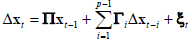,When M0 is used.

 Vector Error Correction Estimates Sample (adjusted): 1981 2007 Included observations: 27 after adjustments Standard errors in ( ) & t-statistics in [ ] Cointegrating Eq: CointEq1 M0Y(-1) 1.000000 INFLATION(-1) -0.56081 (0.14203) [-3.94865] LNW(-1) 0.087525 (0.13977) [ 0.62620] LNWQ(-1) -0.08055 (0.12723) [-0.63314] C -0.03537 Error Correction: D(M0Y) D(INFLATION) D(LNW) D(LNWQ) CointEq1 0.127690 0.736591 0.797174 1.525176 (0.04105) (0.37744) (0.32431) (0.43357) [ 3.11057] [ 1.95153] [ 2.45805] [ 3.51769] D(M0Y(-1)) -0.28127 0.128016 -4.31386 -4.46408 (0.25006) (2.29924) (1.97559) (2.64117) [-1.12480] [ 0.05568] [-2.18358] [-1.69019] D(INFLATION(-1)) -0.01625 0.100973 -1.05449 -1.14141 (0.04674) (0.42972) (0.36923) (0.49362) [-0.34775] [ 0.23498] [-2.85594] [-2.31232] D(LNW(-1)) -0.0502 0.143676 -1.04298 -1.05492 (0.05177) (0.47605) (0.40904) (0.54685) [-0.96948] [ 0.30181] [-2.54983] [-1.92911] D(LNWQ(-1)) -0.00411 0.199097 1.241541 1.643856 (0.04747) (0.43649) (0.37505) (0.50140) [-0.08664] [ 0.45613] [ 3.31036] [ 3.27852] C 0.002253 -0.02813 0.039518 0.017801 (0.00206) (0.01898) (0.01631) (0.02180) [ 1.09135] [-1.48227] [ 2.42321] [ 0.81646] When M1 is used: Vector Error Correction Estimates Sample (adjusted): 1981 2007 Included observations: 27 after adjustments Standard errors in ( ) & t-statistics in [ ] Cointegrating Eq: CointEq1 M1Y(-1) 1.000000 INFLATION(-1) 0.948613 (0.10482) [ 9.04951] LNW(-1) 0.702665 (0.11698) [ 6.00653] LNWQ(-1) -0.519270 (0.10627) [-4.88649] C -0.969343 Error Correction: D(M0Y) D(INFLATION) D(LNW) D(LNWQ) CointEq1 -0.658486 0.304299 -0.261715 0.172338 (0.14373) (0.40421) (0.36018) (0.53732) [-4.58147] [ 0.75283] [-0.72663] [ 0.32074] D(M0Y(-1)) -0.188412 1.224910 -0.329082 1.040162 (0.16964) (0.47709) (0.42512) (0.63420) [-1.11063] [ 2.56746] [-0.77409] [ 1.64011] D(INFLATION(-1)) 0.088606 0.104542 -1.147056 -1.210546 (0.17501) (0.49219) (0.43858) (0.65428) [ 0.50628] [ 0.21240] [-2.61540] [-1.85019] D(LNW(-1)) 0.055913 0.211356 -0.957600 -0.923004 (0.18409) (0.51770) (0.46131) (0.68819) [ 0.30374] [ 0.40826] [-2.07582] [-1.34120] D(LNWQ(-1)) -0.028948 0.126998 1.240329 1.535687 (0.16774) (0.47173) (0.42035) (0.62708) [-0.17258] [ 0.26922] [ 2.95072] [ 2.44893] C -0.006403 -0.022565 0.036992 0.026218 (0.00754) (0.02119) (0.01888) (0.02817) [-0.84977] [-1.06482] [ 1.95897] [ 0.93069]

Table 12: VECM Estimation for [Model 1].

For the general equilibrium [Model 1], the log real wage has a negative effect on real velocity, -0.09 and -0.70 for M0 and M1 respectively. By contrast, the productivity of banking sector has a positive effect on real velocity, 0.08 and 0.52 for M0 and M1 respectively. This result is consistent with the estimates from ECM approach. Hence, it supports the banking approach model in the sense that it is robust various to estimation approaches.

Robustness analysis

A summary of the estimation results are presented in the following Table 13. It collects and compares the results of important estimates by the three estimation approaches to conduct a robustness analysis.

Money Aggregate Estimation Approach [Model 0] [Model 1]
ηmR ηmy ln wt ln wQt
M0 Direct -0.184245 (0.452024) 0.615753 (0.084706) -0.082886 (0.070362) 0.045367 (0.059300)
ECM 0.105070 (0.401988) 1.921966 (0.536143) -0.055594 (0.057830) 0.123902 (0.060345)
VECM 13.91282 (3.297010) 1.194163 (0.148680) -0.087525 (0.139770) 0.080553 (0.127230)
M1 Direct -0.991309 (0.393024) 1.754043 (0.561339) -0.680319 (0.210037) 0.513908 (0.182163)
ECM -2.016102 (0.158939) 1.762568 (0.140245) -0.495504 (0.195712) 0.624849 (0.183561)
VECM -11.62465 (1.168230) 0.557456 (0.039830) -0.702665 (0.116980) 0.519270 (0.106270)

Table 13: Summary of Estimation Results (standard deviation in the parentheses).

In general, [Model 1] is more robust to estimation approach, compared to [Model 0]. In particular, the negative effect of log real wage on real money demand is around -0.06 for M0 and -0.6 for M1, while the positive effect of banking sector productivity is around 0.1 for M0 and 0.5 for M1. Different approaches produce quite similar estimates, which show robustness of [Model 1]. By contrast, the estimates for [Model 0] fluctuate around drastically from -12 to 14, which suggest a probable misspecification of partial equilibrium model.

Moreover, when M1 is used, the results are more robust, compared to the results when M0 is used. For example, the effect of banking sector productivity jumps from 0.045 to 0.124 when M0 is used, while it only wanders around 0.5 with a small deviation when M1 is used. It implies that M1 is more stable than M0.

#### Conclusion

To study the money demand in China, this paper summarizes the latest advances in both theoretical and empirical literature in money demand. An essential distinction between partial equilibrium model and general equilibrium model is emphasized. The fast financial development in China advocates the use of the banking approach DSGE model, which is following the New Classical tradition, in contrast to the New Keynesian model proposed by Dai et al. .

Three estimation approaches are applied to estimate the banking approach general equilibrium model and the results are compared with a benchmark partial equilibrium model. Also, M0 and M1 are used to test the robustness of the results. It shows that partial equilibrium model is still working quite well in explaining the money demand in China. The estimated interest elasticity of money demand is around -1 if M1 is used, but insignificant otherwise. Meanwhile, the income elasticity of money demand ranges from 0.5 to 2.0. In general, the results are not robust to estimation approach. The large volatility in estimation suggests a probable misspecification in the model [13-15].

By contrast, the empirical findings show that the banking approach general equilibrium model is favored in this aspect. The inverse velocity of money is justified to be negatively correlated with real wage around -0.06 for M0 and -0.6 for M1. The productivity of banking sector plays a positive role in determining the inverse velocity of money around 0.1 for M0 and 0.5 for M1. The results show that the general equilibrium model has a better performance in robustness check. Moreover, the results are more stable if M1 is used for both models [16-19].

To conclude, there are two implications of this paper. On the methodology, the general equilibrium model, especially the banking approach, is justified to work better than the traditional partial equilibrium model, in that the former provides a more robust and sensible empirical result. On the practicality, it is shown that fast development in banking sector of China has brought out significantly negative effect on money demand. That is to say, people tend to substitute money demand by banking activities more when the banking sectors are more efficient. Moreover, this negative effect on M1 is relatively greater than that on M0. Hence, the importance of banking sector in understanding money demand in China, or actually in any economy, is emphasized and justified. It was ignored in traditional study of money demand, but can no longer be downplayed nowadays.

#### References

Select your language of interest to view the total content in your interested language

### Article Usage

• Total views: 2296
• [From(publication date):
December-2016 - Jul 18, 2019]
• Breakdown by view type
• HTML page views : 2082Can't read the image? click here to refresh